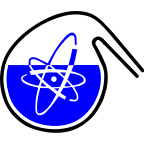#RadChem 2018

13-18 May 2018
Casino Conference Centre
Europe/Prague timezone

## Structure and thermodynamics of the complexes of neptunium (V) with dicarboxylates in aqueous solution

15 May 2018, 11:30
15m
Red Hall (Casino Conference Centre)

### Red Hall

#### Casino Conference Centre

Reitenbergerova 4/95, Mariánské Lázně, Czech Republic
Verbal Separation Methods, Speciation

### Speaker

Mr Martin M. Maiwald (Ruprecht-Karls Universität Heidelberg)

### Description

Introduction
The complexation of actinides with different organic ligands is an important topic in terms of nuclear waste disposal. Depending on the host rock these molecules are either naturally occurring organic compounds as in pore waters of clay rock formations (e.g. acetate, lactate) or model compounds (e.g. oxalate, malonate, succinate) of organic macromolecules (polycarboxylate based cement additives, humic substances) and their degradation products. These compounds may serve as complexing agents towards actinides and thus influence their migration behaviour. A detailed thermodynamic description of these processes is needed for a comprehensive long-term safety assessment of a nuclear waste repository. However, most of the so-far available data is valid only at 25 °C and/or fixed ionic strength.$^{1}$ The thermodynamic functions (log $\beta^{0}_{j}$(T), $\Delta_r$H$^{0}_{m}$, $\Delta_r$S$^{0}_{m}$, $\Delta_r$C$^{0}_{p,m}$) and SIT ion-ion interaction parameters ($\epsilon_T$(i,k)) are often missing. Due to the radioactive decay, elevated temperatures are expected in the near-field of a repository. This will considerably alter the (geo)chemical reactions of the actinides and thermodynamic data of them at increased temperatures are mandatory for a comprehensive thermodynamic model.

Results and Discussion
In this work the complexation of Np(V) with oxalate (Ox$^{2-}$), malonate (Mal$^{2-}$), and succinate (Succ$^{2-}$) is studied as a function of the temperature (20 – 85 °C) and ionic strength (I$_m$(NaCl or NaClO4) = 0.5 – 4.0 mol kg$^{-1}$) using near infrared absorption spectroscopy. The structure of the formed complexes is investigated by extended x-ray absorption fine structure spectroscopy (EXAFS). The formation of the complex species [NpO$_2$(L)$_n$]$^{1-2n}$ (n = 1, 2) is confirmed for all ligands. Application of the specific ion interaction theory (SIT) and the integrated Van´t Hoff equation yields the thermodynamic functions (log $\beta^{0}_{j}$(T), $\Delta_r$H$^{0}_{m}$, $\Delta_r$S$^{0}_{m}$) and the SIT-specific binary ion-ion-interaction parameters ($\epsilon_T$(i,k)) for the different complexation reactions. The results show, that with increasing length of the alkyl chain of the ligand the reaction enthalpy of the complexation reaction shifts from exothermic (Ox$^{2-}$) to endothermic (Mal$^{2-}$, Succ$^{2-}$) and the stability constants decrease by 2.5 – 6 orders of magnitude. The EXAFS data reveal that the change of the thermodynamic properties results from different coordination modes and ring sizes of the formed chelate complexes. Ox$^{2-}$ and Mal$^{2-}$ form 5- and 6-membered chelate rings with Np(V) by coordinating in a side-on mode to Np(V) whereas Succ$^{2-}$ is not capable of forming a chelate ring and coordinates in an end-on fashion.

References
1. Guillaumont, R et al., Update on the Chemical Thermodynamics of Uranium, Neptunium, Plutonium, Americium and Technetium. Elsvier B.V.: 2003.

### Primary author

Mr Martin M. Maiwald (Ruprecht-Karls Universität Heidelberg)

### Co-authors

Dr Daniel R. Fröhlich (Ruprecht-Karls Universität Heidelberg) Dr Jörg Rothe (Institute for Nuclear Waste Disposal) Dr Kathy Dardenne (Institute for Nuclear Waste Disposal) Dr André Rossberg (Helmholtz-Zentrum Dresden Rossendorf) Dr Andrej Skerencak-Frech (Institute for Nuclear Waste Disposal) Prof. Petra J. Panak (Ruprecht-Karls Universität Heidelberg)

### Presentation Materials

There are no materials yet.